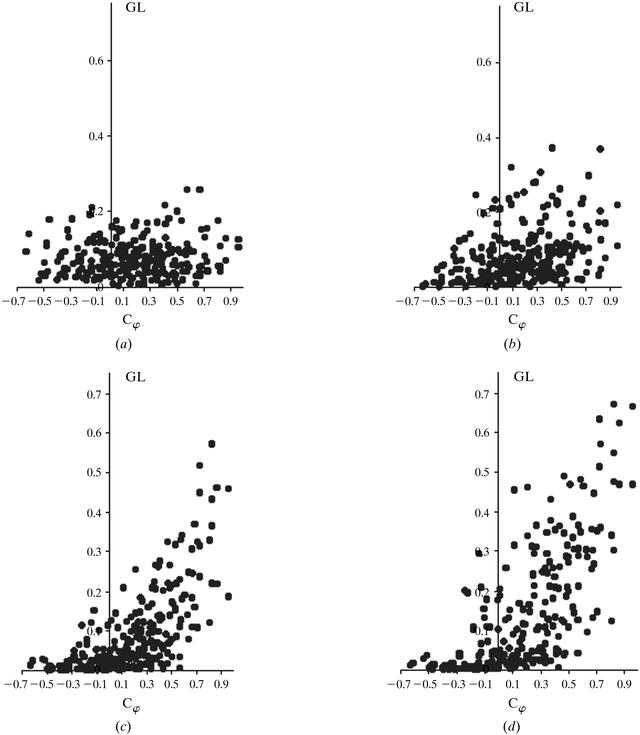disable zoom     view article Figure 5 tRNA–synthetase case. GL phase extension for variants from the first permutation (d = 68 Å). 12 phases were fixed (d = 68 Å) and the phases of six strong reflections (d = 48–68 Å) were permuted. (a) The extension from the variant with Cφ = −0.49. (b) The extension from the variant with Cφ = 0.31. (c) The extension from the variant with Cφ = 0.94. (d) The extension from the variant with Cφ = 0.9997. Cφ was calculated at d = 48–68 Å. GL was calculated at d = 40 Å. The cutoff level was ω = 0.64. The volume of the molecule region was 0.3 of the cell, B = 8000.BIOLOGICALCRYSTALLOGRAPHY
ISSN: 1399-0047
Volume 56| Part 10| October 2000| Pages 1245-1252